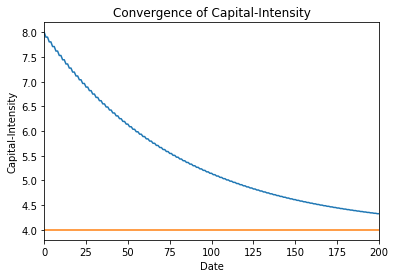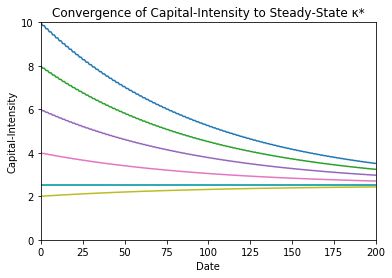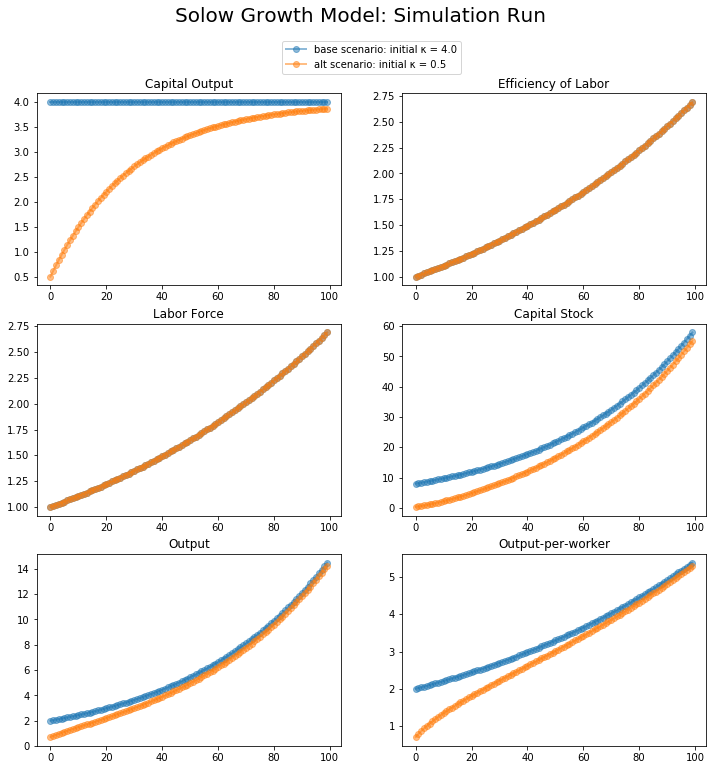## **3. Growing Along and Converging to the Balanced-Growth Equilibrium Path** ¶

### **3.1. The Balanced-Growth Equilibrium Path** ¶

#### **3.1.1. The Balanced-Growth Equilbrium Capital Intensity $\kappa^*$** ¶

We define $\kappa^*$ as that value of capital-intensity $\kappa$ for which, at the current levels of the parameters $n, g, \delta, s$, and $\theta$:

(2.2.10) $\frac{s}{\kappa} - \delta = n + g$

is satisfied. That is true if and only if:

(3.1.1) $\kappa^* = \frac{s}{n+g+\delta}$

If the capital-intensity $\kappa = \kappa^*$, then it is constant. The economy is then in balanced growth.

From (2.1.2) we see that—as capital-intensity $\kappa$ is then constant at the value $\kappa^*$—the proportional growth rate $g_Y$ of total income and production in the economy $Y$ is then equal to n+g, the sum of the growth rate $n$ of the labor force. From (2.1.3) we see that—as capital-intensity $\kappa$ is then constant at the value $\kappa^*$—the proportional growth rate $g_y$ of output per worker is than equal to teh proportional growth rate $g$ of the efficiency of labor. From (2.1.1) we see that the proportional growth rate $g_K$ of the economy's total capital stock is then the same $n + g$ as the growth rate $g_Y$ of income and production $Y$.

#### **3.1.2. Calculating the Balanced-Growth Equilbrium Path** ¶

We can then—if we know the parameter values of the model, the initial values $L_0$ and $E_0$ of the labor force and labor efficiency at some time we index equal to 0, and that the economy is on its balanced-growth equilibrium path—calculate what all variables of interest in the economy will be at any time whatsoever:

Total income and prdouction will be:

(3.1.2) $Y^*_t = \left(\kappa^* \right)^\theta E_t L_t $$= \left(\kappa^* \right)^\theta e^{gt}E_0 e^{nt}L_0$$ = \left(s/(n+g+\delta) \right)^\theta e^{gt}E_0 e^{nt}L_0$

Income and production per worker will be:

(3.1.3) $y^*_t = \left(\kappa^* \right)^\theta E_t $$= \left(\kappa^* \right)^\theta e^{gt}E_0$$ = \left(s/(n+g+\delta) \right)^\theta e^{gt}E_0$

The capital stock will be:

(3.1.4) $K^*_t = \kappa^* Y^*_t$$= \left(s/(n+g+\delta) \right)^{(1+\theta)} e^{gt}E_0 e^{nt}L_0$

The labor force will be:

(3.1.5) $L^*_t = e^{nt}L_0$

And labor efficiency will be:

(3.1.6) $E^*_t = e^{gt}E_0$

### **3.2. Converging to the Balanced-Growth Equilibrium Path** ¶

#### **3.2.1. The Dynamics of Capital Intensity** ¶

But what if $\kappa ≠ \kappa^*$? What happens then? Since $s = \kappa^*(n+g+\delta)$, we can multiply (2.2.9) by $\kappa$ and then rewrite it in terms of the equilibrium capital-intensity $\kappa^*$ as:

(3.2.1) $\frac{d\kappa}{dt} = s/(1+\theta) - (n+g+\delta)\kappa/(1+\theta)$

(3.2.2) $\frac{d\kappa}{dt} = (n+g+\delta)\kappa^*/(1+\theta) - (n+g+\delta)\kappa/(1+\theta)$

(3.2.3) $\frac{d\kappa}{dt} = - \frac{n+g+\delta}{1+\theta} (\kappa-\kappa^*)$

This is of the form of the very first differential equations one encounters in mathematics: it is the exponential equation $dx/dt = k x$, with the constant $k$ here equal to $-(n+g+\delta)/(1+\theta)$. This equation has the solution, if the value of capital-intensity $\kappa$ is known at some time $t=0$ to be $\kappa_0$:

(3.2.4) $\kappa = \kappa^* + e^{-((n+g+\delta)/(1+\theta))t}(\kappa_0 - \kappa^*)$

(3.2.3) holds always, for that moment's values of $n, g, \delta, \theta$ , and $s$, whatever they may be.

(3.2.4) holds only while $n, g, \delta, \theta$ , and $s$ are constant. If any of them change, you then have to recalibrate and recompute, with a new initial value of $\kappa_0$, equal to its value when the model's parameters jumped, and a new and different value of $\kappa^*$.

If $n, g, \delta, \theta$, and $s$are constant or near-constant, then (3.2.4) is a very powerful tool: it tells us that the economy's capital-intensity $\kappa$ follows over time a path of exponential convergence. It is, at time zero, equal to its initial condition $\kappa_0$. It then converges towards its asymptote $\kappa^*$, reducing the gap between its value and $\kappa^*$ at any time t to a fraction $1/e$ of its previous value as of time $t + \Delta_{1/e}t$, where this $1/e$ convergence time is:

(3.2.5) $\Delta_{1/e}t = (n+g+\delta)/(1+\theta)$.

#### **3.2.2. Gaining Intuition About the Convergence of Capital-Intensity to $\kappa^*$** ¶

Immediately below this paragraph are some Python code cells to help you gain some intuition with respect to the dynamics by which the capital-intensity $\kappa$ of an economy following the Solow growth model converges to its balanced-growth equilibrium value $\kappa^*$. Once again, play with the code cells—it is the only way to make the algebra real:

In :
# CODE CELL: CONVERGENCE OF 𝜅 TO 𝜅^*
#
# this code cell plots how the the economy's
# capital-intensity 𝜅 converges to its steady-
# state balanced-growth level 𝜅^* no matter what
# the initial condition 𝜅_0.
#
# either accept the values given below in the block for
# the parameters s, n, g, 𝛿, and θ, and the intitial
# condition on capital-intensity 𝜅_0, plus the time T
# you wish to calculate convergence for, or substitute
# your own preferred values in the relevant code lines
# in the block. then execute this code cell, and see
# what results:

# ----
# BEGIN BLOCK

T = 200

𝜅_0 = 8
s = 0.20
n = 0.01
g = 0.015
𝛿 = 0.025
θ = 1

# END BLOCK
# ----

import numpy as np
import pandas as pd
import matplotlib.pyplot as plt

𝜅_star = s/(n+g+𝛿)
𝜅_max = 2*𝜅_star
𝜅_min = 0.5

𝜅_star_series = [𝜅_star]
𝜅_series = [𝜅_0]

for t in range(T):
𝜅_star_series = 𝜅_star_series + [𝜅_star]
𝜅_series = 𝜅_series + [𝜅_star + (𝜅_series[t-1] - 𝜅_star)*np.exp(-(n+g+𝛿)/(1+θ))]

𝜅_convergence_df = pd.DataFrame()
𝜅_convergence_df['capital_intensity'] = 𝜅_series

ax = plt.gca()

𝜅_convergence_df.capital_intensity.plot(ax=ax)
title = 'Convergence of Capital-Intensity')

ax.set_xlabel("Date")
ax.set_ylabel("Capital-Intensity")

plt.show()In :
# CODE CELL: DEFINITION OF CLASS 𝜅_convergence_graph
#
# this is a reference copy of the 𝜅_convergence_graph
# Python class. it will be kept in the delong_classes
# local file and accessed as:
#
#     delong_classes.𝜅_convergence_graph
#
# use this class to plot how the the economy's
# capital-intensity 𝜅 converges to its steady-
# state balanced-growth level 𝜅^* no matter what
# the initial condition 𝜅_0:

import numpy as np
import pandas as pd
import matplotlib.pyplot as plt

class 𝜅_convergence_graph:

def __init__(self, 𝜅_0 = 3,
s = 0.20,
n = 0.01,
g = 0.015,
𝛿 = 0.025,
θ = 1,
T = 200):
self.𝜅_0, self.s, self.n, self.g, self.𝛿, self.θ, self.T = 𝜅_0, s, n, g, 𝛿, θ, T

def draw(self):
"Draw the convergence graph"
𝜅_0, s, n, g, 𝛿, θ, T = self.𝜅_0, self.s, self.n, self.g, self.𝛿, self.θ, self.T
𝜅_star = s/(n+g+𝛿)
𝜅_max = 2*𝜅_star
𝜅_min = 0.5

𝜅_star_series = [𝜅_star]
𝜅_series = [𝜅_0]

for t in range(T):
𝜅_star_series = 𝜅_star_series + [𝜅_star]
𝜅_series = 𝜅_series + [𝜅_star + (𝜅_series[t-1] - 𝜅_star)*np.exp(-(n+g+𝛿)/(1+θ))]

𝜅_convergence_df = pd.DataFrame()
𝜅_convergence_df['capital_intensity'] = 𝜅_series

ax = plt.gca()

𝜅_convergence_df.capital_intensity.plot(ax=ax)
title = 'Convergence of Capital-Intensity to Steady-State κ*')

ax.set_xlabel("Date")
ax.set_ylabel("Capital-Intensity")

In :
# CODE CELL: USING CLASS 𝜅_convergence_graph TO EXAMINE
# THE CONVERGENCE OF A SOLOW GROWTH MODEL'S CAPITAL-
# INTENSITY 𝜅 TO ITS BALANCED-GROWTH VALUE:
#
#     𝜅^* = s/(n+g+𝛿)
#
# either accept the values given below in the block for
# the parameters s, n, g, 𝛿, and θ, and the intitial
# capital-intensity conditions 𝜅_max and 𝜅_reduce, plus
# the time T you wish to calculate convergence for,
# or, alternatively, you can substitute your own preferred
# values in the relevant code lines found inside of
# the code block. then execute this code cell, and see
# what results:

# ----
# BEGIN BLOCK

𝜅_max = 10
𝜅_reduce = 2
s = 0.15
n = 0.02
g = 0.015
𝛿 = 0.025
θ = 2
T = 200

# END BLOCK
# ----

import delong_classes

plt.cla()

𝜅 = 𝜅_max
for i in range(5):
cg = delong_classes.𝜅_convergence_graph(𝜅_0=𝜅, s = s, n = n,
g = g, 𝛿 = 𝛿, θ = θ, T = T)
cg.draw()
𝜅 = 𝜅-𝜅_reduce

plt.ylim(0, 𝜅_max)
plt.show()#### **3.2.3. The Dynamics of the Other Variables in the Economy** ¶

Given:

(3.2.4) $\kappa = \kappa^* + e^{-((n+g+\delta)/(1+\theta))t}(\kappa_0 - \kappa^*)$

knowledge of the initial values of capital-intensity, the labor force , and the efficiency-of-labor $\kappa_0, L_0,$ and $E_0$; and knowledge of the parameters $s, n, g, \delta$; and $\theta$; we can then immediately calculate the values from time $t = 0$, until some parameter shifts, of all the other variables in the economy from ths equations:

(3.2.6) $Y_t = \left(\kappa_t \right)^\theta E_t L_t = \left(\kappa_t \right)^\theta e^{gt}E_0 e^{nt}L_0$

(3.2.7) $y_t = \left(\kappa_t \right)^\theta E_t = \left(\kappa_t \right)^\theta e^{gt}E_0$

(3.2.8) $K_t = \kappa_t Y_t$

(3.2.9) $L_t = e^{nt}L_0$

(3.2.10) $E_t = e^{gt}E_0$

#### **3.2.4. Gaining Intuition About Convergence and Shocks** ¶

Immediately below this paragraph are some Python code cells to help you gain some intuition with respect to the dynamics by which an economy following the Solow growth model converges to and then follows along its balanced-growth path. The first cell below contains a reference copy of the delong_classes.solow Python class. The second cell below plots a six-panel figure showing the behavior of variables of interest for T periods from an arbitrary starting point. This second cell graphs both a baseline scenario, and an alternative scenario showing what happened after a discontinuous jump in parameters, or what would have happened alternatively had parameters jumped. Once again, play with the code cells—it is the only way to make the algebra real:

In :
# DEFINING THE delong_classes.solow PYTHON CLASS
#
# # this is a reference copy of the 𝜅_convergence_graph
# Python class. it will be kept in the delong_classes
# local file and accessed as:
#
#     delong_classes.solow
#
# use this class to model the dynamic behavior of
# an economy well-described by the Solow growth model:

import numpy as np
import matplotlib.pyplot as plt
%matplotlib inline

class solow:

"""
Implements the Solow growth model calculation of the
capital-output ratio κ and other model variables
using the update rule:

κ_{t+1} = κ_t + ( 1 - α) ( s - (n+g+δ)κ_t )

Built upon and modified from Stachurski-Sargeant
<https://quantecon.org> class **Solow**
<https://lectures.quantecon.org/py/python_oop.html>
"""

def __init__(self, n=0.01,              # population growth rate
s=0.20,              # savings rate
δ=0.03,              # depreciation rate
α=1/3,               # share of capital
g=0.01,              # productivity
κ=0.2/(.01+.01+.03), # current capital-labor ratio
E=1.0,               # current efficiency of labor
L=1.0):              # current labor force

self.n, self.s, self.δ, self.α, self.g = n, s, δ, α, g
self.κ, self.E, self.L = κ, E, L
self.Y = self.κ**(self.α/(1-self.α))*self.E*self.L
self.K = self.κ * self.Y
self.y = self.Y/self.L
self.α1 = 1-((1-np.exp((self.α-1)*(self.n+self.g+self.δ)))/(self.n+self.g+self.δ))
self.initdata = vars(self).copy()

def calc_next_period_kappa(self):
"Calculate the next period capital-output ratio."
# Unpack parameters (get rid of self to simplify notation)
n, s, δ, α1, g, κ= self.n, self.s, self.δ, self.α1, self.g, self.κ
# Apply the update rule
return (κ + (1 - α1)*( s - (n+g+δ)*κ ))

def calc_next_period_E(self):
"Calculate the next period efficiency of labor."
# Unpack parameters (get rid of self to simplify notation)
E, g = self.E, self.g
# Apply the update rule
return (E * np.exp(g))

def calc_next_period_L(self):
"Calculate the next period labor force."
# Unpack parameters (get rid of self to simplify notation)
n, L = self.n, self.L
# Apply the update rule
return (L*np.exp(n))

def update(self):
"Update the current state."
self.κ =  self.calc_next_period_kappa()
self.E =  self.calc_next_period_E()
self.L =  self.calc_next_period_L()
self.Y = self.κ**(self.α/(1-self.α))*self.E*self.L
self.K = self.κ * self.Y
self.y = self.Y/self.L

"Compute the steady state value of the capital-output ratio."
# Unpack parameters (get rid of self to simplify notation)
n, s, δ, g = self.n, self.s, self.δ, self.g
# Compute and return steady state
return (s /(n + g + δ))

def generate_sequence(self, T, var = 'κ', init = True):
"Generate and return time series of selected variable. Variable is κ by default. Start from t=0 by default."
path = []

# initialize data
if init == True:
for para in self.initdata:
setattr(self, para, self.initdata[para])

for i in range(T):
path.append(vars(self)[var])
self.update()
return path

In :
# CODE CELL: SIX-PANEL BASIC SOLOW GROWTH MODEL FIGURE
#
# use this code cell to create a six-panel time-series
# figure of the most interesting economic variables in
# the basic Solow growth model for T periods from an
# arbitrary starting point.
#
# this cell graphs both a baseline scenario, and an
# alternative scenario showing what happened after
# a discontinuous jump in parameters, or what would
# have happened alternatively had parameters jumped.

import numpy as np
import matplotlib.pyplot as plt
%matplotlib inline

import delong_classes

T = 100

s_base = delong_classes.solow(κ=4.0)
s_base.scenario = "base scenario"
s_alt = delong_classes.solow(κ=0.5)
s_alt.scenario = "alt scenario"

figcontents = {
(0,0):('κ','Capital Output'),
(0,1):('E','Efficiency of Labor'),
(1,0):('L','Labor Force'),
(1,1):('K','Capital Stock'),
(2,0):('Y','Output'),
(2,1):('y','Output-per-worker')
}

num_rows, num_cols = 3,2
fig, axes = plt.subplots(num_rows, num_cols, figsize=(12, 12))
for i in range(num_rows):
for j in range(num_cols):
for s in s_base, s_alt:
lb = f'{s.scenario}: initial κ = {s.initdata["κ"]}'
axes[i,j].plot(s.generate_sequence(T, var = figcontents[i,j]),'o-', lw=2, alpha=0.5, label=lb)
axes[i,j].set(title=figcontents[i,j])

#   global legend
axes[(0,0)].legend(loc='upper center', bbox_to_anchor=(1.1,1.3))
plt.suptitle('Solow Growth Model: Simulation Run', size = 20)
plt.show()## **Lecture Notes: The Solow Growth Model** ¶